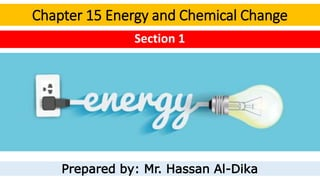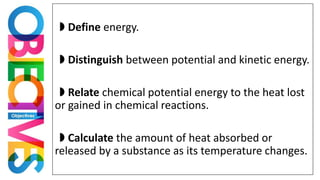Successfully reported this slideshow.

# Energy and chemical change - Section 1×

# Energy and chemical change - Section 1

Introduction to thermochemistry. It includes a definition for heat and energy. the units of heat. How to calculate the heat absorbed or released. Definition of specific heat.

Introduction to thermochemistry. It includes a definition for heat and energy. the units of heat. How to calculate the heat absorbed or released. Definition of specific heat.

### Energy and chemical change - Section 1

1. 1. Chapter 15 Energy and Chemical Change Section 1 Prepared by: Mr. Hassan Al-Dika
2. 2. ◗ Define energy. ◗ Distinguish between potential and kinetic energy. ◗ Relate chemical potential energy to the heat lost or gained in chemical reactions. ◗ Calculate the amount of heat absorbed or released by a substance as its temperature changes.
3. 3. You are probably familiar with the term ENERGY Perhaps you have heard someone say, “I just ran out of energy,” after a strenuous game or a difficult day. Energy-related topics are often discussed in the media.
4. 4. It propels the vehicles that transport youEnergy cooks the food you eat
5. 5. Electric energy provides light and powers devices
6. 6. Energy The ability to do work or produce heat Potential Energy Energy due to the composition or position of an object. Kinetic Energy Energy of motion. It exists in two basic forms:
7. 7. Forms of energy
8. 8. Chemical systems contain both kinetic energy and potential energy. The kinetic energy of a substance is directly related to the constant random motion of its representative particles and is proportional to temperature.
9. 9. • The energy that is stored in a substance because of its composition is called chemical potential energy. • Chemical potential energy plays an important role in chemical reactions. The potential energy of a substance depends on its composition: • the type of atoms in the substance • the number and type of chemical bonds joining the atoms • the particular way the atoms are arranged. Chemical systems contain both kinetic energy and potential energy.
10. 10. Law of conservation of energy/ First law of thermodynamics states that in any chemical reaction or physical process, energy can be converted from one form to another, but it is neither created nor destroyed.
11. 11. When gasoline burns in an automobile’s engine, some of octane’s chemical potential energy is converted to the work of moving the pistons, which ultimately moves the wheels and propels the automobile. However, much of the chemical potential energy of octane is released as heat. Law of conservation of energy/ First law of thermodynamics states that in any chemical reaction or physical process, energy can be converted from one form to another, but it is neither created nor destroyed. The principle component of gasoline is octane (C8H18 ).
12. 12. • It is energy that is in the process of flowing from a warmer object to a cooler object. • The symbol q is used to represent heat. Heat When the warmer object loses energy, its temperature decreases. When the cooler object absorbs energy, its temperature rises.
13. 13. Measuring Heat
14. 14. The joule (J) The SI unit of energy and of heat. Units Calories and Joules A calorie (cal) The amount of energy required to raise the temperature of one gram of pure water by 1°C. 1 joule = 0.2390 calories 1 calorie = 4.184 joules. 1000 calorie = 1 Kcal
15. 15. When your body breaks down sugars and fats to form carbon dioxide and water, these exothermic reactions generate heat that can be measured in Calories. Units 1 nutritional Calorie = 1000 calories = 1 kilocalorie the nutritional Calorie is CAPITALIZED.
16. 16. A breakfast of cereal, orange juice, and milk might contain 230 nutritional Calories. Express this energy in joules. 230 nutritional Calories = 230Kcal = 230000cal 1 𝑐𝑎𝑙 230000 = 4.184 𝑥 X = 9.6 x105 J
17. 17. Compare the specific heat of water and concrete • The specific heat of any substance is the amount of heat required to raise the temperature of one gram of that substance by one degree Celsius. • Because different substances have different compositions, each substance has its own specific heat. Specific Heat
18. 18. Suppose that the temperature of a 5000 g block of concrete sidewalk increased by 6°C. Would it be possible to calculate the amount of heat it had absorbed? Equation for Calculating Heat • q represents the heat absorbed or released. • c represents the specific heat of the substance. • m represents the mass of the sample in grams. • ΔT is the change in temperature in °C • ΔT = T final - T initial .
19. 19. Suppose that the temperature of a 5000 g block of concrete sidewalk increased by 6°C. Would it be possible to calculate the amount of heat it had absorbed? The specific heat of concrete is 0.84 J/(g・°C),
20. 20. For comparison, how much heat would be absorbed by 5000 g of the water when its temperature is increased by 6°C? specific heat of water, 4.184 J/(g°C). If you divide the heat absorbed by the water by the heat absorbed by the concrete , you will find that for the same change in temperature, the water absorbed five times the amount of heat absorbed by the concrete block.

### Editor's Notes

• If the day is especially hot or cold, Energy from burning fuels helps maintain a comfortable temperature in your home and school.
• Electric energy provides light and powers devices from computers and TV sets to cellular phones, MP3 players, and calculators.
Energy was involved in the manufacture and delivery of every material and device in your home.
• In fact, you can think of each cell in your body as a miniature factory that runs on energy derived from the food you eat
• Chemical potential energy For example, the chemical potential energy of propane results from the arrangement of the carbon and hydrogen atoms and the strength of the bonds that join them.
• Propane (C3H8) is an important fuel for cooking and heating. In Figure 15.2b, propane gas combines with oxygen to form carbon dioxide and water. Potential energy stored in the propane bonds is given off as heat.
This is also known as the
When water rushes through turbines in the hydroelectric plant shown in Figure 15.2a, some of the water’s kinetic energy is converted to electric energy.
To better understand the conservation of energy, suppose you have money in two accounts at a bank and you transfer funds from one account to the other. Although the amount of money in each account has changed, the total amount of your money in the bank remains the same. When applied to energy, this analogy embodies the law of conservation of energy. Reading Check State the law of conservation of energy in your own words.
■ Figure 15.2 Energy can change from one form to another but is always conserved.
In a, the potential energy of water is converted to kinetic energy of motion as it falls through the intake from its high position in the reservoir. The rushing water spins the turbine to generate electric energy. In b, the potential energy stored in the bonds of propane molecules is converted to heat.
MAIN Idea Energy can change form and flow, but it is always conserved.
Real-World Reading Link Have you ever watched a roller coaster zoom up and down a track, or experienced the thrill of a coaster ride? Each time a coaster climbs a steep grade or plunges down the other side, its energy changes from one form to another.

In both of these examples, energy changes from one form to another, but energy is conserved— the total amount of energy remains constant.
• The flow of energy and the resulting change in temperature are clues to how heat is measured.
• one nutritional Calorie equals 1000 calories, or one kilocalorie
Note that In the metric system of units, Table 15.1 summarizes the relationships between calories, nutritional Calories, joules, and kilojoules (kJ) and the conversion factors you can use to convert from one unit to another.
For example, one tablespoon of butter contains approximately 100 Calories. This means that if the butter was burned completely to produce carbon dioxide and water, 100 kcal (100,000 cal) of heat would be released.
• Convert Energy Units You must convert nutritional
Calories to calories and then convert calories to joules.
• You have read that one calorie, or 4.184 J, is required to raise the temperature of one gram of pure water by one degree Celsius (1°C). That quantity, 4.184 J/(g・°C), is defined as the specific heat (c) of water.
To raise the temperature of water by one degree Celsius, 4.184 J must be absorbed by every gram of water. Much less energy is required to raise the temperature of an equal mass of concrete by one degree Celsius. You might have noticed that concrete sidewalks get hot during a sunny summer day. How hot depends on the specific heat of concrete, but other factors are also important. The specific heat of concrete is 0.84 J/(g・°C), which means that the temperature of concrete increases roughly five times more than water’s temperature when equal masses of concrete and water absorb the same amount of energy. You can see in
Figure 15.3 that people who have been walking on hot concrete surfaces might want to cool their feet in the water of a fountain.
The cooler waters of the fountain are welcome after walking on the hot concrete sidewalk. The water is cooler because water must absorb five times the number of joules as concrete to reach an equivalent temperature.
Infer How would the temperature change of the concrete compare to that of the water over the course of a cool night.

Recall that the specific heat of a substance tells you the amount of heat that must be absorbed by 1 g of a substance to raise its temperature 1°C. Table 15.2 shows the specific heats for some common substances.
• The specific heat of concrete is 0.84 J/(g・°C), so 1 g of concrete absorbs 0.84 J when its temperature increases by 1°C. To determine the heat absorbed by 5.00 × 1 0 3 g of concrete you must multiply the 0.84 J by 5.00 × 1 0 3 .
Then, because the concrete’s temperature changed by 6.0°C, you must multiply the product of the mass and the specific heat by 6.0°C.
q = c × m × ΔT
The quantity of heat absorbed or released by a substance is equal to the product of its specific heat, the mass of the substance, and the change in its temperature.
• The calculation for q water is the same as it is for concrete except that you must use the specific heat of water, 4.184 J/(g・°C).
You can use this equation to calculate the heat absorbed by the concrete block.
25,000 J or 25 kJ
The total amount of heat absorbed by the concrete block is 25,000 J or 25 kJ.
1.3 × 1 0 5 J or 130 kJ